# Buffers

23 de Feb de 2021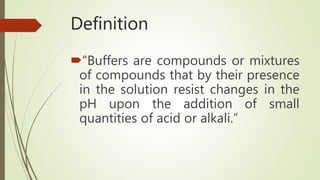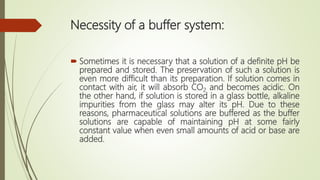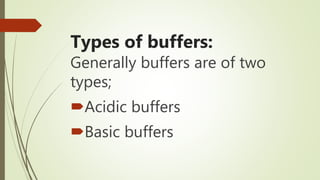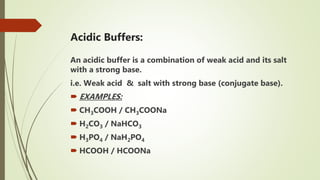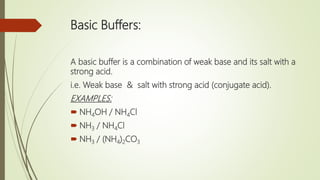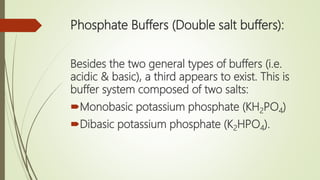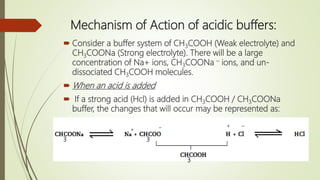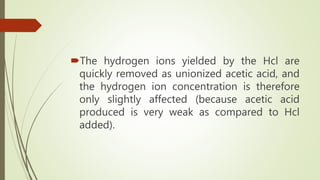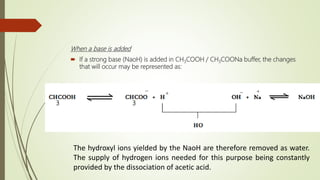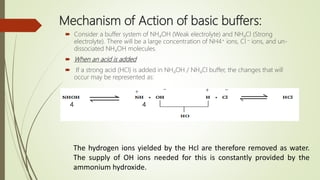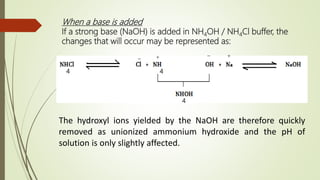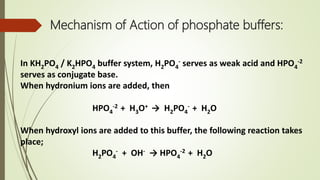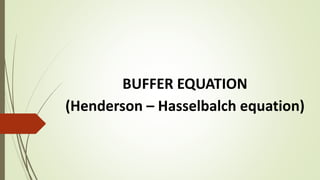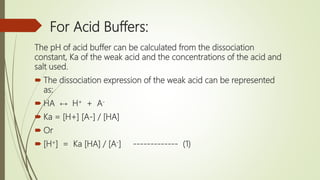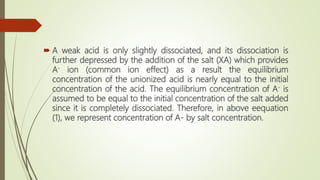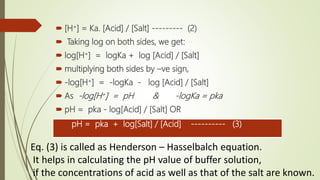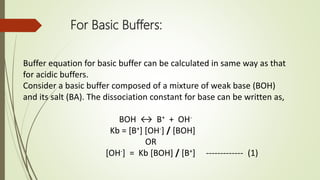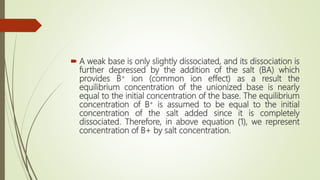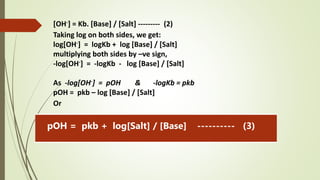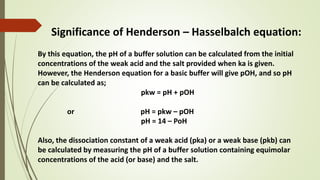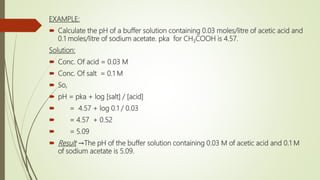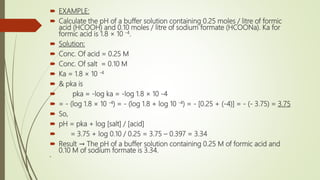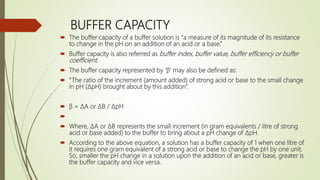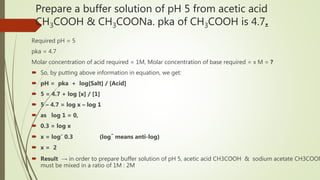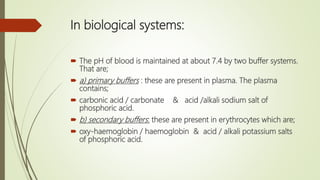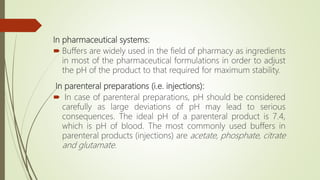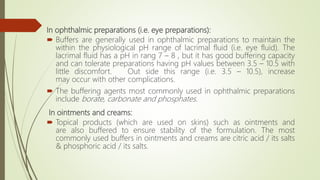1 de 30

### Buffers

• 2. Definition “Buffers are compounds or mixtures of compounds that by their presence in the solution resist changes in the pH upon the addition of small quantities of acid or alkali.”
• 3. Necessity of a buffer system:  Sometimes it is necessary that a solution of a definite pH be prepared and stored. The preservation of such a solution is even more difficult than its preparation. If solution comes in contact with air, it will absorb CO2 and becomes acidic. On the other hand, if solution is stored in a glass bottle, alkaline impurities from the glass may alter its pH. Due to these reasons, pharmaceutical solutions are buffered as the buffer solutions are capable of maintaining pH at some fairly constant value when even small amounts of acid or base are added.
• 4. Types of buffers: Generally buffers are of two types; Acidic buffers Basic buffers
• 5. Acidic Buffers: An acidic buffer is a combination of weak acid and its salt with a strong base. i.e. Weak acid & salt with strong base (conjugate base).  EXAMPLES:  CH3COOH / CH3COONa  H2CO3 / NaHCO3  H3PO4 / NaH2PO4  HCOOH / HCOONa
• 6. Basic Buffers: A basic buffer is a combination of weak base and its salt with a strong acid. i.e. Weak base & salt with strong acid (conjugate acid). EXAMPLES:  NH4OH / NH4Cl  NH3 / NH4Cl  NH3 / (NH4)2CO3
• 7. Phosphate Buffers (Double salt buffers): Besides the two general types of buffers (i.e. acidic & basic), a third appears to exist. This is buffer system composed of two salts: Monobasic potassium phosphate (KH2PO4) Dibasic potassium phosphate (K2HPO4).
• 8. Buffer action The resistance of a buffer solution to a change in pH is known as buffer action.
• 9. Mechanism of Action of acidic buffers:  Consider a buffer system of CH3COOH (Weak electrolyte) and CH3COONa (Strong electrolyte). There will be a large concentration of Na+ ions, CH3COONa – ions, and un- dissociated CH3COOH molecules.  When an acid is added  If a strong acid (Hcl) is added in CH3COOH / CH3COONa buffer, the changes that will occur may be represented as: 3 3 3 + _ + _
• 10. The hydrogen ions yielded by the Hcl are quickly removed as unionized acetic acid, and the hydrogen ion concentration is therefore only slightly affected (because acetic acid produced is very weak as compared to Hcl added).
• 11. When a base is added  If a strong base (NaoH) is added in CH3COOH / CH3COONa buffer, the changes that will occur may be represented as: 3 3 The hydroxyl ions yielded by the NaoH are therefore removed as water. The supply of hydrogen ions needed for this purpose being constantly provided by the dissociation of acetic acid. _ + _ +
• 12. Mechanism of Action of basic buffers:  Consider a buffer system of NH4OH (Weak electrolyte) and NH4Cl (Strong electrolyte). There will be a large concentration of NH4+ ions, Cl – ions, and un- dissociated NH4OH molecules.  When an acid is added  If a strong acid (HCl) is added in NH4OH / NH4Cl buffer, the changes that will occur may be represented as: 4 4 The hydrogen ions yielded by the Hcl are therefore removed as water. The supply of OH ions needed for this is constantly provided by the ammonium hydroxide. + _ + _
• 13. When a base is added If a strong base (NaOH) is added in NH4OH / NH4Cl buffer, the changes that will occur may be represented as: 4 4 4 The hydroxyl ions yielded by the NaOH are therefore quickly removed as unionized ammonium hydroxide and the pH of solution is only slightly affected. + _ + _
• 14. Mechanism of Action of phosphate buffers: In KH2PO4 / K2HPO4 buffer system, H2PO4 - serves as weak acid and HPO4 -2 serves as conjugate base. When hydronium ions are added, then HPO4 -2 + H3O+ → H2PO4 - + H2O When hydroxyl ions are added to this buffer, the following reaction takes place; H2PO4 - + OH- → HPO4 -2 + H2O
• 15. BUFFER EQUATION (Henderson – Hasselbalch equation)
• 16. For Acid Buffers: The pH of acid buffer can be calculated from the dissociation constant, Ka of the weak acid and the concentrations of the acid and salt used.  The dissociation expression of the weak acid can be represented as:  HA ↔ H+ + A-  Ka = [H+] [A-] / [HA]  Or  [H+] = Ka [HA] / [A-] ------------- (1)
• 17.  A weak acid is only slightly dissociated, and its dissociation is further depressed by the addition of the salt (XA) which provides A- ion (common ion effect) as a result the equilibrium concentration of the unionized acid is nearly equal to the initial concentration of the acid. The equilibrium concentration of A- is assumed to be equal to the initial concentration of the salt added since it is completely dissociated. Therefore, in above eequation (1), we represent concentration of A- by salt concentration.
• 18.  [H+] = Ka. [Acid] / [Salt] --------- (2)  Taking log on both sides, we get:  log[H+] = logKa + log [Acid] / [Salt]  multiplying both sides by –ve sign,  -log[H+] = -logKa - log [Acid] / [Salt]  As -log[H+] = pH & -logKa = pka  pH = pka - log[Acid] / [Salt] OR pH = pka + log[Salt] / [Acid] ---------- (3) Eq. (3) is called as Henderson – Hasselbalch equation. It helps in calculating the pH value of buffer solution, if the concentrations of acid as well as that of the salt are known.
• 19. For Basic Buffers: Buffer equation for basic buffer can be calculated in same way as that for acidic buffers. Consider a basic buffer composed of a mixture of weak base (BOH) and its salt (BA). The dissociation constant for base can be written as, BOH ↔ B+ + OH- Kb = [B+] [OH-] / [BOH] OR [OH-] = Kb [BOH] / [B+] ------------- (1)
• 20.  A weak base is only slightly dissociated, and its dissociation is further depressed by the addition of the salt (BA) which provides B+ ion (common ion effect) as a result the equilibrium concentration of the unionized base is nearly equal to the initial concentration of the base. The equilibrium concentration of B+ is assumed to be equal to the initial concentration of the salt added since it is completely dissociated. Therefore, in above equation (1), we represent concentration of B+ by salt concentration.
• 21. [OH-] = Kb. [Base] / [Salt] --------- (2) Taking log on both sides, we get: log[OH-] = logKb + log [Base] / [Salt] multiplying both sides by –ve sign, -log[OH-] = -logKb - log [Base] / [Salt] As -log[OH-] = pOH & -logKb = pkb pOH = pkb – log [Base] / [Salt] Or pOH = pkb + log[Salt] / [Base] ---------- (3)
• 22. Significance of Henderson – Hasselbalch equation: By this equation, the pH of a buffer solution can be calculated from the initial concentrations of the weak acid and the salt provided when ka is given. However, the Henderson equation for a basic buffer will give pOH, and so pH can be calculated as; pkw = pH + pOH or pH = pkw – pOH pH = 14 – PoH Also, the dissociation constant of a weak acid (pka) or a weak base (pkb) can be calculated by measuring the pH of a buffer solution containing equimolar concentrations of the acid (or base) and the salt.
• 23. EXAMPLE:  Calculate the pH of a buffer solution containing 0.03 moles/litre of acetic acid and 0.1 moles/litre of sodium acetate. pka for CH3COOH is 4.57. Solution:  Conc. Of acid = 0.03 M  Conc. Of salt = 0.1 M  So,  pH = pka + log [salt] / [acid]  = 4.57 + log 0.1 / 0.03  = 4.57 + 0.52  = 5.09  Result →The pH of the buffer solution containing 0.03 M of acetic acid and 0.1 M of sodium acetate is 5.09.
• 24.  EXAMPLE:  Calculate the pH of a buffer solution containing 0.25 moles / litre of formic acid (HCOOH) and 0.10 moles / litre of sodium formate (HCOONa). Ka for formic acid is 1.8 × 10 -4.  Solution:  Conc. Of acid = 0.25 M  Conc. Of salt = 0.10 M  Ka = 1.8 × 10 -4  & pka is  pka = -log ka = -log 1.8 × 10 -4  = - (log 1.8 × 10 -4) = - (log 1.8 + log 10 -4) = - [0.25 + (-4)] = - (- 3.75) = 3.75  So,  pH = pka + log [salt] / [acid]  = 3.75 + log 0.10 / 0.25 = 3.75 – 0.397 = 3.34  Result → The pH of a buffer solution containing 0.25 M of formic acid and 0.10 M of sodium formate is 3.34. 
• 25. BUFFER CAPACITY  The buffer capacity of a buffer solution is “a measure of its magnitude of its resistance to change in the pH on an addition of an acid or a base.”  Buffer capacity is also referred as buffer index, buffer value, buffer efficiency or buffer coefficient.  The buffer capacity represented by ‘β’ may also be defined as:  “The ratio of the increment (amount added) of strong acid or base to the small change in pH (ΔpH) brought about by this addition”.  β = ΔA or ΔB / ΔpH   Where, ΔA or ΔB represents the small increment (in gram equivalents / litre of strong acid or base added) to the buffer to bring about a pH change of ΔpH.  According to the above equation, a solution has a buffer capacity of 1 when one litre of it requires one gram equivalent of a strong acid or base to change the pH by one unit. So, smaller the pH change in a solution upon the addition of an acid or base, greater is the buffer capacity and vice versa.
• 26. Prepare a buffer solution of pH 5 from acetic acid CH3COOH & CH3COONa. pka of CH3COOH is 4.7. Required pH = 5 pka = 4.7 Molar concentration of acid required = 1M, Molar concentration of base required = x M = ?  So, by putting above information in equation, we get:  pH = pka + log[Salt] / [Acid]  5 = 4.7 + log [x] /   5 – 4.7 = log x – log 1  as log 1 = 0,  0.3 = log x  x = log- 0.3 (log- means anti-log)  x = 2  Result → in order to prepare buffer solution of pH 5, acetic acid CH3COOH & sodium acetate CH3COON must be mixed in a ratio of 1M : 2M
• 28. In biological systems:  The pH of blood is maintained at about 7.4 by two buffer systems. That are;  a) primary buffers : these are present in plasma. The plasma contains;  carbonic acid / carbonate & acid /alkali sodium salt of phosphoric acid.  b) secondary buffers: these are present in erythrocytes which are;  oxy-haemoglobin / haemoglobin & acid / alkali potassium salts of phosphoric acid.
• 29. In pharmaceutical systems:  Buffers are widely used in the field of pharmacy as ingredients in most of the pharmaceutical formulations in order to adjust the pH of the product to that required for maximum stability. In parenteral preparations (i.e. injections):  In case of parenteral preparations, pH should be considered carefully as large deviations of pH may lead to serious consequences. The ideal pH of a parenteral product is 7.4, which is pH of blood. The most commonly used buffers in parenteral products (injections) are acetate, phosphate, citrate and glutamate.
• 30. In ophthalmic preparations (i.e. eye preparations):  Buffers are generally used in ophthalmic preparations to maintain the within the physiological pH range of lacrimal fluid (i.e. eye fluid). The lacrimal fluid has a pH in rang 7 – 8 , but it has good buffering capacity and can tolerate preparations having pH values between 3.5 – 10.5 with little discomfort. Out side this range (i.e. 3.5 – 10.5), increase may occur with other complications.  The buffering agents most commonly used in ophthalmic preparations include borate, carbonate and phosphates. In ointments and creams:  Topical products (which are used on skins) such as ointments and are also buffered to ensure stability of the formulation. The most commonly used buffers in ointments and creams are citric acid / its salts & phosphoric acid / its salts.